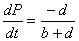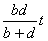Prof. Bryan Caplan

bcaplan@gmu.edu

http://www.bcaplan.com

Econ 849

Week 3: Taxation: Incidence, Efficiency, and Equity

I.                     The Concept of Incidence

A.                 The concept of incidence is arguably the most profound one in economics.  Economic shocks (including economic policies) frequently "ripple out" in counter-intuitive ways, as Bastiat patiently explains in "What Is Seen and What is not Seen."

B.                 Common sense says that:

1.                  Farmers benefit from rain.

2.                  Thieves profit from stealing.

3.                  The poor benefit from the minimum wage.

4.                  Employers pay the cost of safety regulation.

C.                But economists usually think that:

1.                  Rain benefits consumers.

2.                  Thieves earn only their opportunity cost.

3.                  The minimum wage hurts many of the poor.

4.                  Employees pay for safety.

II.                   Incidence and Elasticity

A.                 But what exactly determines incidence?

B.                 The surprising answer is (supply and demand) elasticity.  Inelastic factors bear burdens and enjoy windfalls; elastic factors tend to avoid both.

C.                Intuition: Elastic factors exit if conditions get worse, and enter if conditions get better.  Therefore they largely avoid the effect of shocks.  Inelastic factors, in contrast, stay put and "suck it up."

D.                Caveat emptor: In real-world applications, you must often look carefully ("upstream" and "downstream") for hidden elastic or inelastic factors.

1.                  Ex: Property taxation

III.                  Tax Incidence and Elasticity, I: The Suppliers' Viewpoint

A.                 The logic of incidence (and the irrelevance of written law) is easiest to see for excise taxes in simple two-equation S&D models.  Definitions:

1.                  P=observed price tag

2.                  PS=price paid to suppliers

3.                  PD=price paid by demanders

4.                  t=per-unit tax

B.                 Assume:

1.                  S: Q=a+bPS

2.                  D: Q=c-dPD

C.                The law says that "sellers have to pay the government a tax of t per unit sold."  The tax is thus "included in the price."

D.                Then:

1.                  PD=P

2.                  PS=P-t

E.                 Substitute in for PS and PD to get:

1.                  S: Q=a+b(P-t)

2.                  D: Q=c-dP

F.                 Solve for P: P=[-a+c+bt]/[b+d]

G.                So:.  (We can convert from slopes to elasticities by multiplying top and bottom by t/P).

II.                   Taxation and Incidence, II: The Demanders' Viewpoint

B.                 Suppose instead that the law says that "consumers have to pay the government a tax of t per unit sold."  The tax is thus "added onto the price."

C.                Then:

1.                  PD=P+t

2.                  PS=P

D.                Substitute in for PS and PD to get two equations in 2 unknowns:

1.                  S: Q=a+bP

2.                  D: Q=c-d(P+t)

E.                 Solve for P: P=[-a+c-dt]/[b+d]

F.                 And what is (P+t)?  P+t=[-a+c-dt]/[b+d] + t =[-a+c+bt]/[b+d]!

G.                So:, and.

H.                 Exercise: Prove that Q falls by [bd/b+d]t either way.

I.                     Applications: What if b=0?  ¥?  What if d=0?  ¥?

A.                 A costlessly collected lump-sum tax has no deadweight costs.

B.                 Virtually all other taxes do because people use real resources and change their behavior to avoid paying.

C.                In fact, taxes can have deadweight costs even if they don't change observed behavior!  How?  By changing behavior relative to the response to lump-sum taxation.

D.                Ex: Income taxation.  A lump sum tax decreases wealth and therefore normally increases hour devoted to work.  But a proportional income tax that raises the same revenue also reduces the marginal incentive to work.  If the income and substitution effects cancel, a deadweight loss remains.

1.                  Thought experiment: How many more hours would you work if additional hours suddenly became tax-free?

A.                 Because of these complexities, economists often switch from consumer surplus to more complex measures of consumer welfare.  But we'll stick with CS for simplicity.  Just remember that CS understates deadweight loss when income effects are significant.

B.                 Returning to our two-equation system, revenue raised is the rectangle tQ.

C.                But market participants lose more than tQ because they buy and sell less to avoid taxes.

D.                How much more?  Consumers' DW loss is; sellers DW loss is.  Total:.

E.                 Substitutingforreveals that total DW loss is.

1.                  Again, we can convert to elasticities by multiplying through by t/P.

V.                 The Laffer Curve

A.                 The Laffer curve is a simple extension of the basic economics of taxation:  How does revenue depend on tax rate?  It will NOT be linear, because taxes change incentives.

B.                 Standard observation: revenues are \$0 at a 0% tax (mathematically), and presumably fall to \$0 at a 100% rate (since there is no incentive to work).

C.                Where is the revenue-maximizing point on the Laffer curve?  Empirically, it is a tough question, and sensitive to time horizon.

D.                In a simple 2-equation framework, though, getting the answer is fairly easy.  Just differentiate tQ(t) wrt t and find t*.

E.                 Answer: t*=[bc+ad]/[2bd].  Note that if a perfectly inelastic resource exists, there is no finite solution.

F.                 Exercise: Prove that a tax-maximizing government will produce an even smaller quantity than a profit-maximizing monopolist.

G.                People have occasionally said that the peak of the Laffer curve is somehow the "optimal" tax rate.  But this essentially assumes a lexiocographic preference for government revenues over all other goods.

VI.               The Ramsey Rule

A.                 Imagine that a government wants to raise a given amount of revenue with the minimum DW loss.  How must this be done?  The philosopher Frank Ramsey worked out the answer - now known as the "Ramsey rule" - in the 1920's.

B.                 Given proportional taxation, linear (and independent) demand, and constant MC of production, the Ramsey rule tells us to set tax rates inversely proportional to demand elasticities.  The more elastic the good, the less it is taxed.

1.                  If the elasticity of corn is .3, and the elasticity of grapes is .6, the Ramsey rule tells us to set the tax rate on corn equal to twice the tax rate on grapes.

C.                Under these assumptions, all quantities consumed fall by equal fractions.

D.                There is an enormous literature that relaxes the preceding assumptions in predictable ways.  Ramsey's intuition is quite robust.

E.                 If there is a fixed cost of taxing an item at all, DW loss minimization is likely to prescribe an exclusive focus on low-elasticity items.

VII.              Normative Optimal Taxation Versus Positive Optimal Taxation

A.                 In the previous lecture, I pointed out two controversial inferences many draw from public goods theory:

1.                  Normative PGT: If something is a public good, government should provide it or subsidize it.

2.                  Positive PGT:  Goods provided by governments are, at least in large part, public goods.

B.                 There are two parallel inferences (both controversial) that many draw from optimal taxation theory (especially the Ramsey rule):

1.                  Normative OTT: Governments should raise revenue in accordance with optimal tax theory.

1.                  Positive OTT: Governments do in fact raise revenue in accordance with optimal tax theory

D.                Variant: Governments should and/or do use OTT, but balance it against the benefits of reducing inequality.

VIII.            Landsburg's Perfect Tax

A.                 In Fair Play, Landsburg offers some interesting thoughts on tax equity and efficiency.

B.                 Almost all taxation is based on actual earnings, but to avoid disincentives, you must base it on potential earnings.

C.                But potential earnings are not directly observable.  So the trick is to find observable predictors of potential earnings which will not themselves vary with incentives.

1.                  Ex: Natural versus realized beauty

D.                Some interesting extensions:

1.                  Leisure taxation

2.                  Race-based taxation

3.                  Gender-based taxation

IX.               Egalitarianism, Rawls, and Tax Equity

A.                 Most people, including most economists, see market-created inequality as a serious problem and want to use tax policy to combat it.

B.                 Why?  There are a wide variety of arguments.  Among economists, perhaps the most popular comes from assuming that everyone has roughly equivalent cardinal utility functions, and that these functions exhibit decreasing marginal utility of wealth.

1.                  With costless lump-sum taxation, utilitarianism then implies perfect equality.  (Without lump-sum taxation, of course, utilitarianism prescribes some income inequality).

C.                The philosopher John Rawls worked out a popular non-utilitarian case for strong - but not full - egalitarianism.  For Rawls, equality is a morally privileged starting point.    But that Pareto improvements away from full equality are OK.  If increasing inequality benefits even the worst-off, then it is morally acceptable.  Otherwise not.

X.                 Landsburg, Rothbard, and Tax Equity

A.                 There are other economists, however, who question the whole goal of "reducing inequality."

B.                 Landsburg has both "practical" and "principled" objections to his "Perfect Tax."

1.                  Practical: Endogeneity of taxation.  Low DW loss probably means very high taxes and a lot of wasteful government spending.

2.                  Principled: Redistribution is inconsistent.  Almost no one advocates international, intertemporal, or non-material redistribution.

C.                Rothbard makes the simple point that arguing about "justice in taxation" is rather silly if taxation is itself unjust.  "Equality of unjust treatment can never be upheld as an ideal of justice."

D.                Rothbard goes on to critique almost all of the main "canons of justice" in taxation:

1.                  Tax exemptions are not morally equivalent to subsidies.

2.                  "The 'ability-to-pay' principle resembles more the highwayman's principle of taking where the taking is good."

3.                  Benefit principle doesn't justify progressive or even proportional taxation.